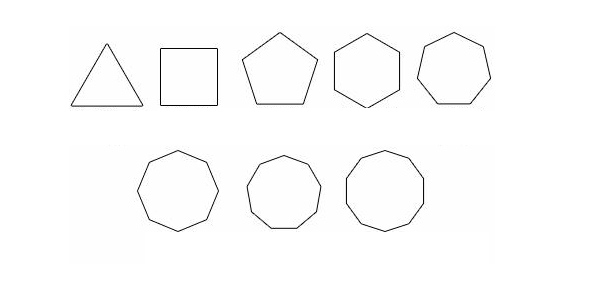# Define Polygons

14 QuestionsSettingsMatch the polygon to its meaning.

Related Topics
• 1.
A five sided polygon is a
• A.

Pentagon

• B.

Hexagon

• C.

Heptagon

• 2.
A seven sided polygon is a
• A.

Pentagon

• B.

Hexagon

• C.

Heptagon

• 3.
A six sided polygon is a
• A.

Pentagon

• B.

Hexagon

• C.

Heptagon

• 4.
An eight sided polygon is a
• A.

Decagon

• B.

Nonagon

• C.

Octagon

• 5.
A nine sided polygon is a
• A.

Dodecagon

• B.

Decagon

• C.

nonagon

• 6.
A ten sided polygon is a
• A.

Decagon

• B.

Nonagon

• C.

Dodecagon

• 7.
A twelve sided polygon is a
• A.

Decagon

• B.

Octagon

• C.

Dodecagon

• 8.
A polygon has three or more sides and equal number of angles.
• A.

True

• B.

False

• 9.
A polygon is a closed figure made by joining line segments, where each line segment intersects exactly two others.
• A.

True

• B.

False

• 10.
The figure is a poylgon if it is not a closed figure:
• A.

True

• B.

False

• 11.
If a line that contains a side of a polygonalso contains a point inside the polygon, the polygon is this
• A.

Interior angle

• B.

Exterior angle

• 12.
This is on the inside of a polygon, formed by its sides.
• A.

Regular

• B.

Irregular

• C.

Concave

• 13.
This is formed by a sde of a polygon and an extension of an adjacent side.
• A.

Irregular angle

• B.

Exterior angle

• C.

Interior angle

• 14.
This describes a polygon in which all sides are congruent and all angles are congruent.
• A.

Convex

• B.

Irregular

• C.

Regular